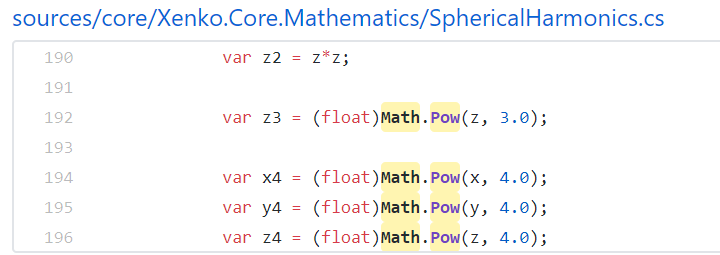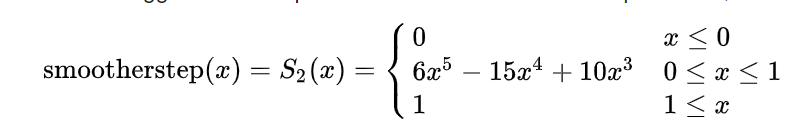# Optimize Math.Pow(x, c) where c is 2, 1, -1 or 0 #31978

Open
wants to merge 8 commits into
from
Open

# Optimize Math.Pow(x, c) where c is 2, 1, -1 or 0#31978

wants to merge 8 commits into from
+205 −0

## Conversation

Contributor

### EgorBo commented Feb 8, 2020 • edited

Resurrects dotnet/coreclr#26552
Optimizes:

```Math.Pow(x,  2) --> x*x
Math.Pow(x,  1) --> x
Math.Pow(x, -1) --> 1/x```

(same for `MathF` and `float`)

This time it's done in the `importer.cpp` and handles all kinds of the first argument (introduces a temp variable if needed, e.g. for `GT_CALL`).

#### Example:

```static double Pow2(double x)  => Math.Pow(x, 2);
static double Pow1(double x)  => Math.Pow(x, 1);
static double PowN1(double x) => Math.Pow(x, -1);```

#### Current codegen:

```; Method Tests:Pow2(double):double
vzeroupper
vmovsd   xmm1, qword ptr [reloc @RWD00]
jmp      System.Math:Pow(double,double):double

; Method Tests:Pow1(double):double
vzeroupper
vmovsd   xmm1, qword ptr [reloc @RWD00]
jmp      System.Math:Pow(double,double):double

; Method Tests:PowN1(double):double
vzeroupper
vmovsd   xmm1, qword ptr [reloc @RWD00]
jmp      System.Math:Pow(double,double):double```

#### New codegen:

```; Method Tests:Pow2(double):double
vzeroupper
vmulsd   xmm0, xmm0, xmm0
ret

; Method Tests:Pow1(double):double
vzeroupper
ret  ; just return xmm0

; Method Tests:PowN1(double):double
vzeroupper
vmovsd   xmm1, qword ptr [reloc @RWD00]
vdivsd   xmm0, xmm1, xmm0
ret```

It seems this pattern can be found in gamedev, e.g.. Xenko (a game engine): https://github.com/xenko3d/xenko/search?q=Math.Pow&unscoped_q=Math.Pow
Also the dotnet/performance benchmarks use it: https://github.com/dotnet/performance/blob/8aed638c9ee65c034fe0cca4ea2bdc3a68d2a6b5/src/benchmarks/micro/runtime/Burgers/Burgers.cs
Jitdiff for bcl:

``````Total bytes of diff: -13 (-0.00% of base)
diff is an improvement.

Top file improvements by size (bytes):
-13 : System.Private.CoreLib.dasm (-0.00% of base)

1 total files with size differences (1 improved, 0 regressed), 107 unchanged.

Top method improvements by size (bytes):
-9 (-2.05% of base) : System.Private.CoreLib.dasm - System.Globalization.CalendricalCalculationsHelper:DefaultEphemerisCorrection(int):double
-4 (-0.73% of base) : System.Private.CoreLib.dasm - System.Globalization.CalendricalCalculationsHelper:EquationOfTime(double):double

Top method improvements by size (percentage):
-9 (-2.05% of base) : System.Private.CoreLib.dasm - System.Globalization.CalendricalCalculationsHelper:DefaultEphemerisCorrection(int):double
-4 (-0.73% of base) : System.Private.CoreLib.dasm - System.Globalization.CalendricalCalculationsHelper:EquationOfTime(double):double

2 total methods with size differences (2 improved, 0 regressed), 261780 unchanged.
``````

The optimization can be extended to handle more cases once some sort of fast-math mode appears in .NET Core.

added 3 commits Feb 7, 2020
``` Optimize Math(F).Pow(X, C) when C is `0, 1, -1, 2` ```
``` 1c1ca76 ```
``` Fix pow_cns.cs test ```
``` ad05394 ```
``` Don't introduce a tmp for GT_CNS_DBL ```
``` dc2b301 ```
added 2 commits Feb 8, 2020
``` remove Pow(x, 0), add a test for floats passed by ref ```
``` ee22b6c ```
``` Add tests for side-effects ```
``` 54378fa ```
Member

### benaadams commented Feb 8, 2020

 If you can do it at import with consts, would it be worth going higher? e.g. to 5 in you linked example 3-4 crops upFor 5 I was going to suggest smootherstepHowever, you'd probably write it like `x * x * x * (x * (x * 6 - 15) + 10)`
Contributor Author

### EgorBo commented Feb 8, 2020 • edited

 @benaadams If I understand you correctly I can't optimize other constants in "safe math" mode, e.g. Math.Pow(x, 4) can be optimized to ``` vmulsd xmm0, xmm0, xmm0 vmulsd xmm0, xmm0, xmm0``` (a single xmm0 register!) but it might return a slightly different value (and violate the ieee754 spec) see https://godbolt.org/z/R78Ev-
added 3 commits Feb 8, 2020
``` Fix tests ```
``` 267280c ```
``` tmp commit (CI test) ```
``` 6c4307f ```
``` Math.Pow(NaN, 2) might return a "different" NaN than NaN*NaN ```
``` 316ad79 ```
Member

### stephentoub commented Feb 8, 2020

Contributor Author

### EgorBo commented Feb 9, 2020

 CI failures are unrelated (#31985)
reviewed
 @@ -4226,6 +4226,39 @@ GenTree* Compiler::impMathIntrinsic(CORINFO_METHOD_HANDLE method, op1 = nullptr; if (intrinsicID == CORINFO_INTRINSIC_Pow && impStackTop().val->IsCnsFltOrDbl())

#### tannergooding Feb 11, 2020

Member

Are there cases we are going to miss by handling this in the importer, rather than later in morph or lowering?

#### EgorBo Feb 11, 2020

Author Contributor

@tannergooding not sure honestly, but "inlining" cases are handled, e.g.

```float Foo(float x, float y) => MathF.Pow(x, y);

float Test(float x) => Foo(x, 2.0f);```

`Test` will be optimized.

#### EgorBo Feb 11, 2020

Author Contributor

```float Foo(float x, float y) => MathF.Pow(x, y + 1.0f);
float Test(float x) => Foo(x, 1.0f);```

(constant folding after inlining)

reviewed
 // Math.Pow(x, 2) -> x*x impPopStack(); GenTree* arg0 = impPopStack().val; if (arg0->OperIs(GT_LCL_VAR, GT_CNS_DBL))

#### EgorBo Feb 14, 2020

Author Contributor

hm.. looks like I actually need `fgMakeMultiUse` here